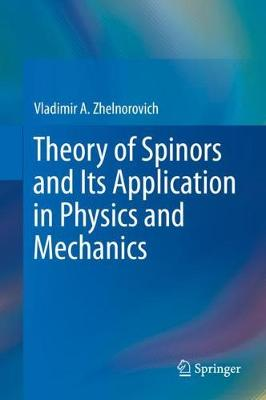•# Theory of Spinors and Its Application in Physics and Mechanics (Hardback)

(author)
£109.99
Hardback 392 Pages / Published: 06/11/2019
• We can order this

Usually dispatched within 3 weeks

This book contains a systematic exposition of the theory of spinors in finite-dimensional Euclidean and Riemannian spaces. The applications of spinors in field theory and relativistic mechanics of continuous media are considered.

The main mathematical part is connected with the study of invariant algebraic and geometric relations between spinors and tensors. The theory of spinors and the methods of the tensor representation of spinors and spinor equations are thoroughly expounded in four-dimensional and three-dimensional spaces. Very useful and important relations are derived that express the derivatives of the spinor fields in terms of the derivatives of various tensor fields.

The problems associated with an invariant description of spinors as objects that do not depend on the choice of a coordinate system are addressed in detail. As an application, the author considers an invariant tensor formulation of certain classes of differential spinor equations containing, in particular, the most important spinor equations of field theory and quantum mechanics. Exact solutions of the Einstein-Dirac equations, nonlinear Heisenberg's spinor equations, and equations for relativistic spin fluids are given.

The book presents a large body of factual material and is suited for use as a handbook. It is intended for specialists in theoretical physics, as well as for students and post-graduate students of physical and mathematical specialties.

Publisher: Springer Nature Switzerland AG
ISBN: 9783030278359
Number of pages: 392
Weight: 776 g
Dimensions: 235 x 155 mm
Edition: 1st ed. 2019